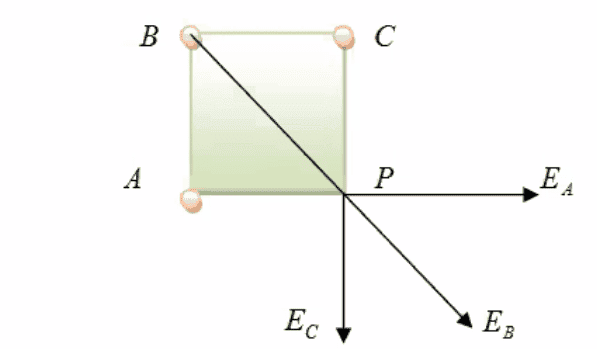# Electric field due to three point charges

• emmanual
In summary, the conversation discusses calculating the intensity of three point charges and determining the resultant intensity using the parallelogram law. The individual electric field vectors are expressed in rectangular coordinates and the challenge lies in adding them up component-wise to find the net electric field. It is suggested to find the separate electric fields first and then add them together for simplicity. f

#### emmanual

Homework Statement
Three point charges q1(+3C), q2(+4C), q3(+3C) are located in a square-shaped form with a distance of 2cm with a 90° cone in every edge(picture in the attached file 39png). Calculate the resultant intensity of point P.
Relevant Equations
Coulomb's law :
F=q1q2/4πεr2
Parallelogram law:
R=√(P²+ Q²+ 2PQcosα)
I've calculated the intensity for every point charge which are
EA = 6.741 x 10¹³ NC¯¹
E
B = 4.494 x 10¹¹ NC¯¹
E
C = 6.741 x 10¹³ NC¯¹
and I am pretty sure about this far but I am struggling to calculate the X-axis intensity and Y-axis intensity to find the entire approximate intensity with the parallelogram law.#### Attachments

Welcome to PF.It looks like you have a good start, but those electric field values should be vectors so that you can do the vector sum at P. Can you express all 3 of them as vectors in rectangular coordinates at P so that you can add them up component-wise?

Welcome to PF.It looks like you have a good start, but those electric field values should be vectors so that you can do the vector sum at P. Can you express all 3 of them as vectors in rectangular coordinates at P so that you can add them up component-wise?
I've already expressed all 3 in vectors in the first file w the arrows on p and identified them with the EA, EB and EC and which I used to express the resultant intensity too and Idk how I'm supposed to add it up that's where I'm stucklike I know it's supposed to be 2 values, not 3 and IDK how to reduce it into 2 values which will be valid mathematically

Are you saying YDK how to decompose a vector into its components? The magnitude of the vector is represented as the hypotenuse of a right triangle with the x and y components as the two right sides. Have you not seen this before?

•SammyS and berkeman
to keep things simple, find separate electrics field of all the charges and in the end add them to get a net electric field due to all the charges, if you want to do other way around its up to you.